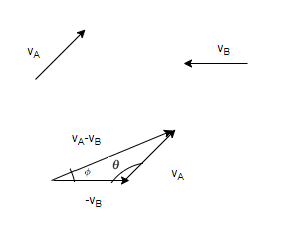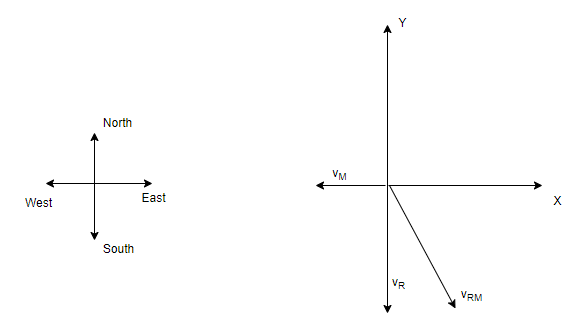# Relative velocity in Two Dimension

## Relative velocity in Two Dimensions

• we have already seen the Relative Velocity in 1D motion.Lets see how to define it in two -dimensional motion
• For two objects A and B moving with the uniform velocities $\vec{V_A}$ and $\vec{V_B}$
Relative velocity is defined as
$\vec{V_{BA}} =\vec{V_B} - \vec{V_A}$
where $\vec{V_{BA}}$ is relative velocity of B relative to A

Similarly relative velocity of A relative to B
$\vec{V_{AB}} =\vec{V_A} - \vec{V_B}$
Therefore
$\vec{V_{BA}} = - \vec{V_{AB}}$
• we will need to add or subtracting components along x & y direction to get the relative velocity
Suppose
$\vec{V_A}=v_{xa} \hat{i} + v_{ya} \hat{y}$
$\vec{V_B}=v_{xb} \hat{i} + v_{yb} \hat{y}$

Relative velocity of B relative to A
$\vec{V_{BA}} =v_{xb} \hat{i} + v_{yb} \hat{y} -(v_{xa} \hat{i} + v_{ya} \hat{y})$
$=\hat{i}(v_{xb} -v_{xa}) + \hat{j}(v_{yb} - v_{ya})$

• For three dimensions motion
$\vec{V_A}=v_{xa} \hat{i} + v_{ya} \hat{y} + v_{za} \hat{k}$
$\vec{V_B}=v_{xb} \hat{i} + v_{yb} \hat{y} + v_{zb} \hat{k}$

Relative velocity of B relative to A
$\vec{V_{BA}} =v_{xb} \hat{i} + v_{yb} \hat{y} + v_{zb} \hat{k} -(v_{xa} \hat{i} + v_{ya} \hat{y} + v_{za} \hat{k})$
$=\hat{i}(v_{xb} -v_{xa}) + \hat{j}(v_{yb} - v_{ya}) +\hat{k}(v_{zb} - v_{za})$
• Magnitude of the Relative velocity from A to B and B to A is same
$|\vec{V_{BA}}|=|\vec{V_{AB}}|$
• Lets take two vector $\vec{v_A}$ and $\vec{v_B}$ as shown below and find the relative velocity using the law of triangle$|\vec {v_{AB}}|= \sqrt { v_A^2 + v_A^2 -2v_A v_B cos \theta}$
$\tan \phi= \frac {v_A sin \theta}{v_B - v_A cos \theta}$
• Relative velocity concept is very useful when finding the direction of falling and analyzing the direction of swimmer in the river

## Relative motion between Man and Rain

• Here
$\vec{v_m}$ is the velocity of Man with respect to ground
$\vec{v_r}$ is the velocity of Rain with respect to ground
Velocity of Rain with respect to ground will be given by
$\vec{v_{rm}}= \vec{v_r} - \vec{v_m}$
or
$\vec{v_r}= \vec{v_{rm}} + \vec{v_m}$
• So if we are given the velocity of Rain with respect to man and Velocity of Man, then we can find the velocity of rain by doing vector addiotion of both the given velocity
and if we are given the velocity of Rain with respect to ground and Velocity of Man, then we can find the velocity of rain with respect to man by doing vector subtraction of both the given velocity
• We can do these vector addition or subtraction either using component method or the triangle law

Example
Rain is falling vertically with a speed of 35 m/s . A man rides a bicycle with a speed of 12 m/s in east to west direction. What is the direction in which he should hold his umbrella ?
Solution
Here velocity of Rain is given and velocity of man is given and we need to find the velocity of rain with respect to man.
Lets take component method and take x axis along west -east and y axis as vertical ,then$\vec{v_m}=- 12 \hat{i}$
$\vec{v_r}= -35 \hat{j}$
Now
$\vec{v_{rm}}=\vec{v_r} - \vec{v_m}= 12\hat{i} -35\hat{j}$
Angle with Horizontal =$\tan ^{-1} \frac {35}{12}=71^0$

## Relative motion between swimmer and River

• Here
$\vec{v_m}$ is the velocity of Man with respect to ground
$\vec{v_r}$ is the velocity of River with respect to ground
Velocity of Man with respect to river will be given by
$\vec{v_{mr}}= \vec{v_m} - \vec{v_r}$
or
$\vec{v_m}= \vec{v_{mr}} + \vec{v_r}$
• So if we are given the velocity of Man with respect to River and Velocity of River, then we can find the velocity of man by doing vector addition of both the given velocity
and if we are given the velocity of Man with respect to ground and Velocity of River, then we can find the velocity of Man with respect to River by doing vector subtraction of both the given velocity
• We can do these vector addition or subtraction either using component method or the triangle law